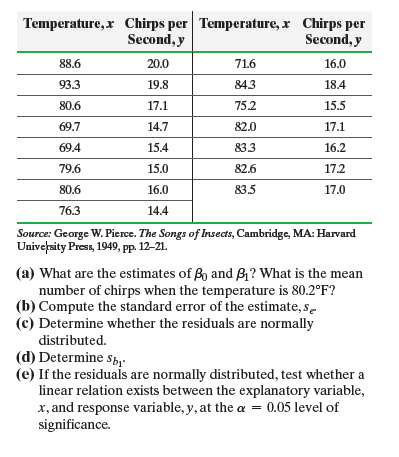×
Get Full Access to Statistics: Informed Decisions Using Data - 5 Edition - Chapter 14 - Problem 2
Get Full Access to Statistics: Informed Decisions Using Data - 5 Edition - Chapter 14 - Problem 2

×

# ?Crickets make a chirping noise by sliding their wings rapidly over each other. Perhaps you have noticed that the number of chirps seems to increase wiISBN: 9780134133539 240

## Solution for problem 2 Chapter 14

Statistics: Informed Decisions Using Data | 5th Edition

• Textbook Solutions
• 2901 Step-by-step solutions solved by professors and subject experts
• Get 24/7 help from StudySoup virtual teaching assistantsStatistics: Informed Decisions Using Data | 5th Edition

4 5 1 257 Reviews
14
1
Problem 2

Crickets make a chirping noise by sliding their wings rapidly over each other. Perhaps you have noticed that the number of chirps seems to increase with the temperature. The following table lists the temperature (in degrees Fahrenheit, °F) and the number of chirps per second for the striped ground cricket.

(a) What are the estimates of b0 and b1? What is the mean number of chirps when the temperature is 80.2°F?

(b) Compute the standard error of the estimate, se. (c) Determine whether the residuals are normally distributed.

(d) Determine sb1

(e) If the residuals are normally distributed, test whether a linear relation exists between the explanatory variable, x, and response variable, y, at the a = 0.05 level of significance

f) If the residuals are normally distributed, construct a 95% confidence interval for the slope of the true leastsquares regression line.

(g) Construct a 90% confidence interval for the mean number of chirps found in part (a).

(h) Predict the number of chirps on a day when the temperature is 80.2°F.

(i) Construct a 90% prediction interval for the number of chirps found in part (h).

(j) Explain why the predicted number of chirps found in parts (a) and (h) are the same, yet the intervals are different.Step-by-Step Solution:

Step 1 of 5) Crickets make a chirping noise by sliding their wings rapidly over each other. Perhaps you have noticed that the number of chirps seems to increase with the temperature. The following table lists the temperature (in degrees Fahrenheit, °F) and the number of chirps per second for the striped ground cricket. (a) What are the estimates of b0 and b1 What is the mean number of chirps when the temperature is 80.2°F (b) Compute the standard error of the estimate, se. (c) Determine whether the residuals are normally distributed. (d) Determine sb1 (e) If the residuals are normally distributed, test whether a linear relation exists between the explanatory variable, x, and response variable, y, at the a = 0.05 level of significance f) If the residuals are normally distributed, construct a 95% confidence interval for the slope of the true leastsquares regression line. (g) Construct a 90% confidence interval for the mean number of chirps found in part (a). (h) Predict the number of chirps on a day when the temperature is 80.2°F. (i) Construct a 90% prediction interval for the number of chirps found in part (h). (j) Explain why the predicted number of chirps found in parts (a) and (h) are the same, yet the intervals are different.

Step 2 of 2

## Discover and learn what students are asking

Calculus: Early Transcendental Functions : Product and Quotient Rules and Higher-Order Derivatives
?Using the Product Rule In Exercises 1–6, use the Product Rule to find the derivative of the function. $$h(t)=\sqrt{t}\left(1-t^{2}\right)$$

Chemistry: The Central Science : Molecular Geometry and Bonding Theories
?In the hydrocarbon (a) What is the hybridization at each carbon atom in the molecule? (b) How many \(

Unlock Textbook Solution